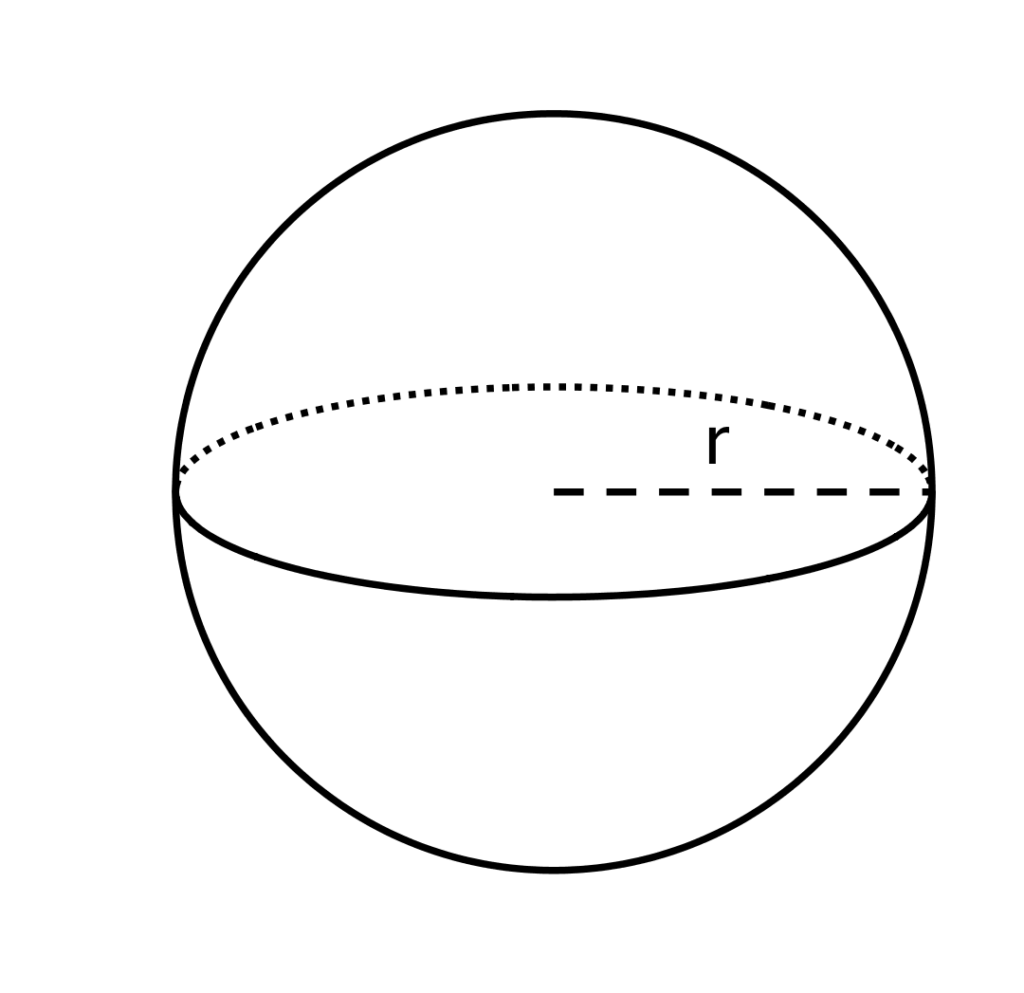# C Program To Find Volume of Sphere | C Programs

C program to calculate the volume of a sphere – In this particular article, we will brief in on the several ways to calculate the volume of a sphere in C programming.

Check out the blog for the suitable examples and sample programs. The compiler has been added as well so that you can check the results yourself.

The methods used to calculate the volume of a sphere in C programming are as follows:

• Using Standard Method
• Using Function
• Using Pointers
• Using Macros

As we all know, a sphere is a 3-dimensional figure made up of infinite points which are equidistant from a single point in the middle. That single point is regarded as the centre of the sphere.

The distance between the surface of the sphere and the centre is regarded as the radius of the sphere. It is an essential component to calculate the volume of a sphere.

A sphere looks like this:As you can see, the radius of the sphere is denoted by r.

The volume of a sphere can be calculated with this formula:

V = 4/3 * pi * r^3

Using this formula itself, here are the various methods to calculate the volume of a sphere in C programming:

## Using Standard Method

1)We have the formula for the volume of a sphere is v=(4*22*r*r)/(7*3);

2)scanf(“%f”,&r) ,scanf function reads the r value, r will be stored into the variable “r”.

3)The r value will substitute into the formula, the calculated value will be store into v, v value will be displayed

using printf(“VOS:%f\n”,v);

output:

### Using Function

1)we are calling the function using volume(r).

2)The called function float volume(float r) will calculate the volume and return the value to v=volume(r).

3)v hold the return value.

output:

#### Using Pointers

1)We are calling the function by passing addresses using volume(&r,&v).

2)volume(float *r,float *v) having pointer variables r,v. This function calculates the volume of the sphere and the value will store into variable v.

3)Using printf statement v value will be displayed.

output:
##### Using Macros

1)The symbolic name to the formula (4*22*r*r*r)/(7*3) is area(r)

2)area(r) replaced by  the expression given at #define

output:

x

## C Program To Check If Alphabet, Digit or Special Character | C Programs

C program to check for an alphabet, digit or special character – In this article, ...